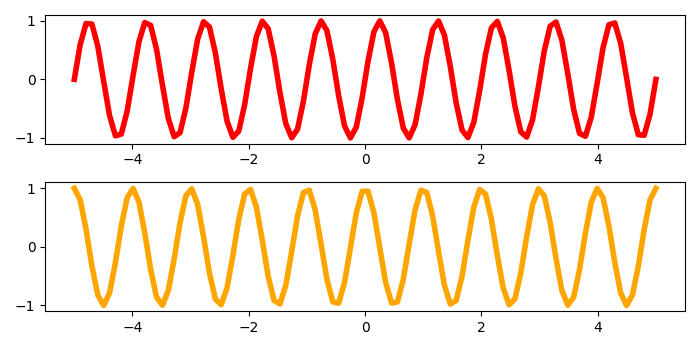# How to set same scale for subplots in Python using Matplotlib?

To set the same scale for subplot in Python using Matplotlib, we can take the following steps −

• Set the figure size and adjust the padding between and around the subplots.
• Create a new figure or activate an existing figure.
• Add an 'ax1' to the figure as part of a subplot arrangement with nrows=2, ncols=1 and index=1.
• Add another axis 'ax2' to the figure as part of a subplot arrangement with nrows=2, ncols=1 and index=2, with shared X-axis (to set same scale for subplots)
• Create "t" data points to plot sine and cosine curves on axes ax1 and ax2.
• To display the figure, use show() method.

## Example

import matplotlib.pyplot as plt
import numpy as np

# Set the figure size
plt.rcParams["figure.figsize"] = [7.00, 3.50]
plt.rcParams["figure.autolayout"] = True

# Plot the figure
fig = plt.figure()

ax2 = fig.add_subplot(2, 1, 2, sharex=ax1)

# Create data points
t = np.linspace(-5, 5, 100)

# Plot sine and cosine curves on ax1 and ax2
ax1.plot(t, np.sin(2 * np.pi * t), color='red', lw=4)
ax2.plot(t, np.cos(2 * np.pi * t), color='orange', lw=4)

plt.show()

## Output

It will produce the following output